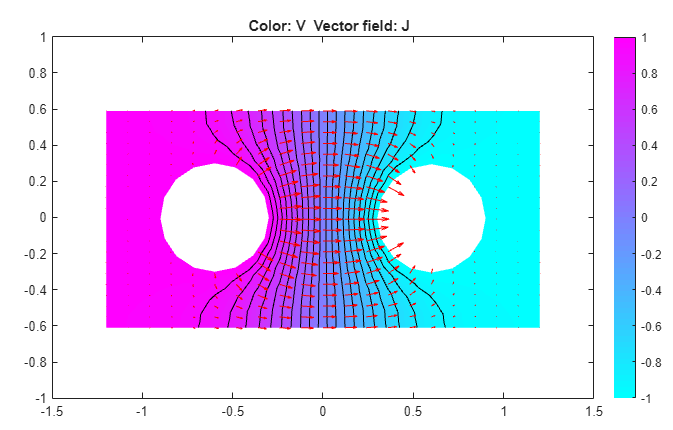## Current Density Between Two Metallic Conductors: PDE Modeler App

Two circular metallic conductors are placed on a brine-soaked blotting paper which serves as a plane, thin conductor. The physical model for this problem consists of the Laplace equation

–∇ · (σV) = 0

for the electric potential V and these boundary conditions:

• V = 1 on the left circular conductor

• V = –1 on the right circular conductor

• the natural Neumann boundary condition on the outer boundaries

`$\frac{\partial V}{\partial n}=0$`

The conductivity is σ = 1.

To solve this equation in the PDE Modeler app, follow these steps:

1. Model the geometry: draw the rectangle with corners at (-1.2,-0.6), (1.2,-0.6), (1.2,0.6), and (-1.2,0.6), and two circles with a radius of 0.3 and centers at (-0.6,0) and (0.6,0). The rectangle represents the blotting paper, and the circles represent the conductors.

```pderect([-1.2 1.2 -0.6 0.6]) pdecirc(-0.6,0,0.3) pdecirc(0.6,0,0.3)```
2. Model the geometry by entering `R1-(C1+C2)` in the Set formula field.

3. Set the application mode to Conductive Media DC.

4. Specify the boundary conditions. To do this, switch to the boundary mode by selecting Boundary > Boundary Mode. Use Shift+click to select several boundaries. Then select Boundary > Specify Boundary Conditions.

• For the rectangle, use the Neumann boundary condition with ```g = 0``` and `q = 0`.

• For the left circle, use the Dirichlet boundary condition with ```h = 1``` and `r = 1`.

• For the right circle, use the Dirichlet boundary condition with ```h = 1``` and `r = -1`.

5. Specify the coefficients by selecting PDE > PDE Specification or clicking the PDE button on the toolbar. Specify `sigma = 1` and `q = 0`.

6. Initialize the mesh by selecting Mesh > Initialize Mesh.

7. Refine the mesh by selecting Mesh > Refine Mesh.

8. Improve the triangle quality by selecting Mesh > Jiggle Mesh.

9. Solve the PDE by selecting Solve > Solve PDE or clicking the = button on the toolbar. The resulting potential is zero along the y-axis, which, for this problem, is a vertical line of antisymmetry.10. Plot the current density J. To do this:

1. Select Plot > Parameters.

2. In the resulting dialog box, select the Color, Contour, and Arrows options.

3. Set the Arrows value to ```current density```.

The current flows, as expected, from the conductor with a positive potential to the conductor with a negative potential. The conductivity σ is isotropic, and the equipotential lines are orthogonal to the current lines.